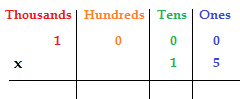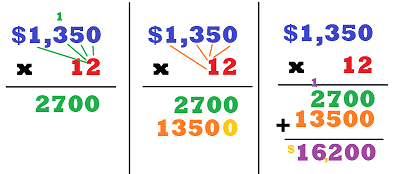# 4 Digit by 2 Digit Multiplication

Instructor: Christine Quist

Christine has taught 4th-5th grade, has worked as a Paraprofessional for Adult Learners, and has a master's degree in Curriculum and Instruction for Elementary Education.

Multiplication plays a very important role in math. But, we wouldn't get very far if we only ever multiplied one digit numbers. In this lesson, we will learn how to multiply four digit numbers by two digit numbers. Don't worry, it is easier than it sounds!

## Big Money

Congratulations on your new job! Although your boss told you how much you would make every month, you want to know how much you will make in a year, so you need to multiply your monthly income, a four digit number, by 12, the number of months in a year. Let's see how to do that!

## The Method

Before we get started, let's review the rules of multiplying multi-digit numbers. First, write the numbers vertically, with the larger number on top of the smaller number. Second, the numbers must be lined up by place value. The ones place in the smaller number needs to be directly under the ones place in the larger number. 1000 x 15 would look like this:Now that we have the rules, let's set up our income problem. Let's say you walk all the dogs in your neighborhood and earn a total of \$1,350 every month for 12 months. Your problem will be \$1,350 x 12. Take a look at the image.

### Multiplication - Round OneBecause you are multiplying by a two digit number, you will multiply through two times. But let's just look at the first round for now.

For the first round, we will be using the 2 in 12 (forget all about the 1 in the 12 for now). The 2 multiplies through the whole four digit number starting in the ones place, then moving to the tens, hundreds and ending at the thousands.

Start with 2 times 0 which equals 0 and place it in the ones place. Next is 2 times 5 which equals 10, place the 0 down below and carry over the 1. 2 times 3 is next which equals 6 plus the carried 1. And last is 2 times 1 in the thousands place, which equals 2.

### Multiplication - Round Two

Now we will use the number 1 in the 12, BUT, because the number you are now using to multiply is in the tens place, there will be no ones; so, you MUST place a 0 in the ones place of the answer (shown by the yellow 0). If you do not place that 0, you will get the wrong answer every time. The 1 will be now be multiplied with each of the four digits of \$1,350.

Now that we have multiplied by all the digits in our bottom number, we will add the two answers together to form the final answer which is \$16,200.

To unlock this lesson you must be a Study.com Member.

### Register to view this lesson

Are you a student or a teacher?

#### See for yourself why 30 million people use Study.com

##### Become a Study.com member and start learning now.
Back
What teachers are saying about Study.com

### Earning College Credit

Did you know… We have over 200 college courses that prepare you to earn credit by exam that is accepted by over 1,500 colleges and universities. You can test out of the first two years of college and save thousands off your degree. Anyone can earn credit-by-exam regardless of age or education level.